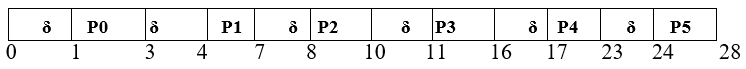FCFS Scheduling with Overhead: In this tutorial, we are going to learn what is overhead in First Come First Served Scheduling (FCFS) algorithm?
Submitted by Monika Jha, on November 10, 2019

In the FCFS scheduling, we consider that all the processes or jobs are the CPU bound jobs (if any process spends most of its time simply using the CPU or doing calculations, so this process is called CPU bound process) only. We do not think about the context switching time.

A procedure in which a processor (central processing unit) follows to change from one task (or job) to another while ensuring that the tasks do not conflict by the context or state of a process so that it can be reloaded when required and execution can be resumed from the same point as earlier, this is called Context Switching.

The average waiting time of the system will be increased If we consider the time taken by the scheduler in context switching. This condition also affects the efficiency of the system. It is always overhead in scheduling.

If we consider the context switching time in the system, so the efficiency will be affected. We can describe it with the following example.

Here, we are considering six processes with process id as P1, P2, P3, P4, P5, and P6. The arrival time and Burst time of these processes are as under,

Process Id Arrival time Burst time
P002
P113
P222
P335
P446
P554

We denote the context switching time by δ sign and let's take δ=1 unit; Then the Gantt chart of the system will be prepared as follows:Gantt Chart

By this overhead, the system will take an extra 1 unit of time after the execution of every process or task to schedule the next task.

```Useless time / Wasted time          = 6 * δ = 6 * 1 = 6 unit
Total time taken by all processes   = 28 unit
Useful time of processes            = 28 unit – 6 unit = 22 unit

Efficiency (η) of system    = Useful time  / Total time
= 22 unit / 28 unit
= 0.7857
= 78.57 %

Inefficiency    = Wasted time / Total time
= (6 / 28 * 100) %
= 21.42 %
```

References:

What's New

Top Interview Coding Problems/Challenges!

Languages: » C » C++ » C++ STL » Java » Data Structure » C#.Net » Android » Kotlin » SQL
Web Technologies: » PHP » Python » JavaScript » CSS » Ajax » Node.js » Web programming/HTML
Solved programs: » C » C++ » DS » Java » C#
Aptitude que. & ans.: » C » C++ » Java » DBMS
Interview que. & ans.: » C » Embedded C » Java » SEO » HR
CS Subjects: » CS Basics » O.S. » Networks » DBMS » Embedded Systems » Cloud Computing
» Machine learning » CS Organizations » Linux » DOS
More: » Articles » Puzzles » News/Updates